# Regularization in BN

This is an introduction of the ICLR 2019 paper Towards Understanding Regularization in Batch Normalization. This was written in Chinese.

# 浅谈深度学习：归一化中的正则与泛化

Ping Luo, Xinjiang Wang, Wenqi Shao

## 1. BN与正则

### 1.1 一个简单例子

import numpy as np
N=10000; M=32
a=np.random.randn(M, N) #随机生成N批长度为M的标准高斯分布的数据
b=(a-np.mean(a, axis=0))/np.std(a, axis=0) #利用批统计量做归一化
print(np.mean((b-a)**2)) #输出平均误差


### 1.3 “隐式”正则的形式：PN+Gamma衰减

$$\ell(\hat{h}^{j}) = \ell(\bar{h}^{j}) + \frac{\partial \ell(\bar{h}^{j})}{\partial \bar{h}^j}(\hat{h}^j-\bar{h}^j)+\frac{1}{2}\frac{\partial^2 \ell(\bar{h}^{j})}{\partial^2 \bar{h}^j}(\hat{h}^j-\bar{h}^j)^2 +\mathcal{O}\big((\hat{h}^j-\bar{h}^j)^2\big)$$

$$=\ell(\bar{h}^{j}) + (A’(\bar{h}^j)-y^j)(\hat{h}^j-\bar{h}^j)+\frac{1}{2}A”(\bar{h}^j)\cdot(\hat{h}^j-\bar{h}^j)^2 +\mathcal{O}\big((\hat{h}^j-\bar{h}^j)^2\big)$$

$$\mathbb{E}_{\mu_{\mathcal{B}},\sigma_{\mathcal{B}}} \big[ (\hat{h}^j-\bar{h}^j)^2 \big]\approx (\frac{\mathbf{w}^T\mathbf{x}-\mu_{\mathcal B}}{\sigma_{\mathcal{B}}})^2\frac{\rho+2}{4M}\gamma^2 + \frac{1}{M}\big(1+\frac{3(\rho+2)}{4M}\big)\gamma^2$$

$$L\approx \frac{1}{P} \sum_{j=1}^{P} \ell(\bar{h}^{j}) + \zeta(h) \gamma^2,~~~ \zeta(h)=\underbrace{\frac{\rho+2}{8M}F_\gamma}_{\mathrm{from~} \sigma_{\mathcal{B}}}+\underbrace{\frac{\mu_{d^2A}}{2M}}_{\mathrm{from~} \mu_{\mathcal{B}}}.$$

### 1.4 特定激活函数的正则形式

ReLU函数：此处我们利用连续的SoftPlus函数来替代ReLU函数来逼近 $f(\bar h)=\lim_{t\rightarrow \infty}\frac{1}{t}(1+\exp(t\bar h))$。可以得到 $A”(\bar h)=f’(\bar h)=\lim_{t\rightarrow\infty}\sigma (t\bar h)=\mathrm{H}(\bar h)$。其中 $\sigma(x) = \frac{1}{1+\exp(-x)}$ 为Sigmoid函数，$H(x)=(\mathrm{sign}(x)+1)/2$ 为Heaviside阶跃函数。所以对于ReLU激活函数，可以得到 $\zeta(h)=\frac{\rho+2}{8M}F_\gamma+\frac{1}{2M}\mathrm{\bar{H} (\bar{h})}$，其中 $\mathrm{\bar{H} (\bar{h})}=\frac{1}{P}\sum_{j=1}^P{\mathrm{\bar{H} (\bar{h}^j)}}$ 代表所有样本的平均被激活值。因此若网络使用ReLU，Gamma衰减可近似为 $\zeta(h)={\frac{\rho+2}{8M}F_\gamma}+{\frac{1}{2M}\frac{1}{P}\sum_{j=1}^P\sigma(\bar{h}^j)}$。

Identity函数：当激活函数为Identity函数 $f(\bar h)=\bar h$ 时，$\zeta(h)$的形式会更加简洁。此时 $\mu_{d^2A}=\frac{1}{P}\sum_{j=1}^P{\bar{h}^j}$。若同时假设全局统计量 ${\bar h}\sim\mathcal{N}(0,1)$，即 $\mu_\mathcal{P}=0$ 和 $\sigma_\mathcal{P}=1$。其损失函数的正则项系数 $\zeta \approx\frac{3}{4M}$，与隐含层取值 $h$ 无关。值得注意的是在做线性回归时一般损失函数定义为 $\ell(\mathbf x)=(\phi(\mathbf x)-y)^2$，所以对其而言 $A”(\bar h)=2$，因此 $\zeta=\frac{3}{2M}$ (对应文章开头最简单的例子)。另外如果网络中无偏置项则无需统计 $\mu_\mathcal B$。这时 $\mu_\mathcal B$ 对正则项的影响可忽略，得到 $\zeta=\frac{1}{2M}$。

### 1.5 正则的意义

• 首先，通过BN的正则化过程，BN的“隐式”正则可以显式表示为PN与Gamma衰减。PN使用全局统计量来代替BN中的随机统计量，使训练过程的随机性变为决定性。PN与正则项的搭配让我们可以使用传统方法例如动态系统和统计物理来理解BN的训练过程和泛化能力。 PN与参数归一化 (WN) 有紧密联系。WN的表达式为 $\nu\frac{\mathbf{w}^T \mathbf{x}^j}{\Vert\mathbf{w}\Vert}$。对比其与PN的形式可以看出当输入服从高斯分布且协方差矩阵为以 $a$ 为对角元素的对角阵时 $\bar{h}^j=\gamma\frac{\mathbf{w}^T \mathbf{x}^j-\mu_\mathcal{P}}{\sigma_\mathcal{P}}+\beta=v\frac{\mathbf{w}^T \mathbf{x}^j}{\Vert\mathbf{w}\Vert}+b$，这里 $v=\gamma/a$ 同时 $b=-\frac{\gamma\mu_\mathcal{P}}{a\Vert \mathbf{w}\Vert_2}+\beta$。

• 其次，Gamma衰减的系数 $\zeta(h) = \frac{\rho+2}{8M}F_\gamma + \frac{1}{2M}\mu_{d^2A}$ 包括两项，但它们来源有所不同。第一项 $\frac{\rho+2}{8M}F_\gamma$ 来源于 $\sigma_{\mathcal B}$ 的噪声，其中 $F_\gamma$ 为Hessian矩阵的对角项。由于 $F_\gamma = \mathbb E[(\frac{\partial \ell}{\partial \gamma})^2]$，因此此项等效为——惩罚损失函数反向传播过程中过大的梯度值以及避免在优化中掉入过于陡峭的局部最优点。当考虑多个神经元或多层网络时该项实际减少不同参数之间的相关性 (co-adaptation)，可类比dropout的作用。 而第二项 $\frac{1}{2M}\mu_{d^2A}$ 来源于 $\mu_{\mathcal B}$，且在激活函数为ReLU时形式化为 $\frac{1}{2M}\frac{1}{P}\sum_{j=1}^P\sigma(\bar{h}^j)$。它代表了数据经过本神经元被激活的概率。因此其等效的作用是在一个隐藏层的神经元中惩罚有过大激活值的神经元，从而降低网络输出值对单一神经元的依赖。为此当输入出现一定噪声或者任一神经元被剪枝后对网络的输出不会造成太大影响。

• 另外，值得注意的是本文分析的正则是施加在 $\gamma$ 上的，也就是BN中的缩放系数。但是，在一些软件实现过程中如果BN后面是ReLU，也往往可以不需要这一缩放系数5。这样一来BN的正则效果就加在了后面卷积层的核函数上。

## 2. BN与泛化

### 2.3 解析解与数值解对比

SGD网络：普通SGD网络定义为 $\phi(x)=\mathbf{w}^T\mathbf{x}$。此时 $\mathbf{w}$ 收敛的解较易得到，其形式即为Moore-Penrose伪逆解 $\mathbf{w} =\left(\mathbf{x} ^T \mathbf{x} \right)^{+}\mathbf{x} ^T {y}$。对比求解过程中未知数个数 $N$ 和方程个数 $P$，我们不难发现当 $\alpha=P/N<1$ 时其解为欠定，而当 $\alpha>1$ 时其解过定。而当 $\alpha=1$ 时解虽然唯一，但是在极小的扰动 $\varepsilon$ 下得到的解不稳定甚至发散。利用随机矩阵理论我们可以得到 $\Sigma$ 在不同 $\alpha$ 值时的特征值分布，从而得到其泛化误差 $$\epsilon_{\mathrm{gen}}^{\mathrm{sgd}}=\begin{cases} 1-\alpha+{\alpha\delta^{2}}{/(1-\alpha)}, & \left(\alpha<1\right)\\ {\delta^{2}}{/(1-\alpha)}. & \left(\alpha>1\right) \end{cases}$$ 若取 $\delta^2=0.25$ 时其泛化误差随 $\alpha$ 的关系可见下图中蓝线部分。当 $\alpha\in[0,1]$ 以及 $\alpha\gg1$ 时泛化误差随 $\alpha$ 增加而减少，体现在随着训练样本数目增加，模型能更好地学习参数。但是当 $\alpha\approx1$ 时输入的协方差矩阵 $\Sigma=\mathbf{x}^T\mathbf x$ 的特征值在 $0$ 处的分布出现峰值，所以这时其伪逆会极不稳定。也就是说当网络试图去拟合教师网络中的噪声 $\varepsilon$，这时训练会出现很强的过拟合。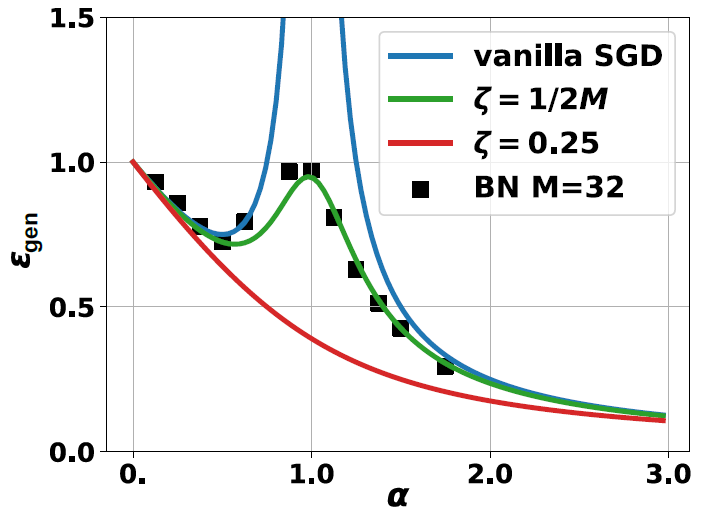BN网络：BN学生网络的形式为 $\phi(\mathbf{x})=\gamma \frac{\mathbf{w}^T\mathbf{x}-\mu_\mathcal{B}}{\sigma_\mathcal{B}}+\beta$。类似教师网络，我们合并偏置项即 $\beta=0$。在BN的数值实验中我们给定输入维度为 $N=4096$ 以逼近热力学极限 (实际上 $N>100$ 左右已足够)。而 $\alpha$ 的值则依据 $\alpha=P/N$ 中的 $P$ 变化决定。在网络优化过程中我们令批次大小 $M=32$ 以观察BN对网络训练的正则效果。其学习得到的泛化误差在上图中标记为实心方块。其趋势也和普通SGD类似，但这时网络的泛化能力得到了显著的改善。特别地当 $\alpha=1$ 时网络不再发散，因为BN的正则效果使激活 $\gamma$ 不致过大。由前面的分析我们得知BN的引入导致网络对 $\gamma$ 有一个隐含的约束，而当输入满足标准高斯分布时其峰度 $\rho=0$。这时不难算出Gamma衰减的 $\zeta(h)$ 可以简化为 $\zeta=\frac{1}{2M}$ 且与 $h$ 无关。

WN与正则网络：根据上文的分析在当前的设定条件下BN网络可以等价于WN+Gamma衰减。所以我们定义 $\phi(\mathbf x) = \gamma \frac{\mathbf{w}^T\mathbf{x}}{\Vert \mathbf{w}\Vert}+\beta$，同BN一样这里偏置项 $b$ 也合并。与此同时在WN的损失函数 $L$ 后面加上Gamma衰减 $\zeta\Vert \gamma \Vert_2^2$。这里的 $\zeta$ 可以任意取值。例如取 $\zeta=0.25$ 时泛化误差曲线效果最好如上图红线，此时刚好抵消教师网络中的噪声。

### 2.4 CNN中的正则效果

#### 2.4.1 PN+Gamma衰减与BN等效？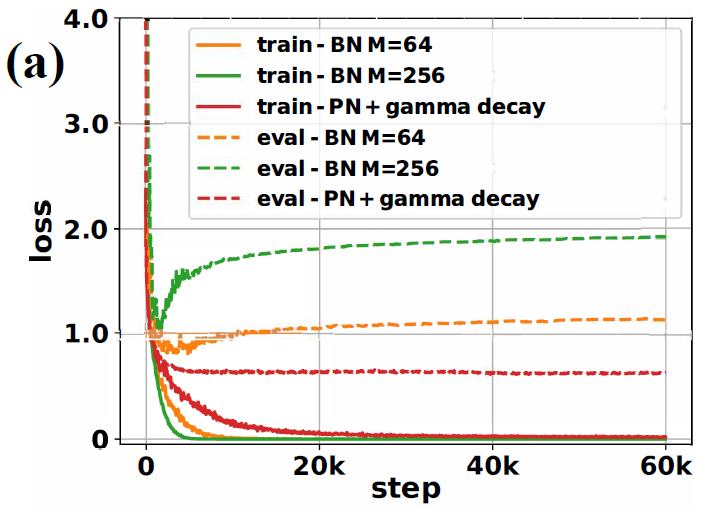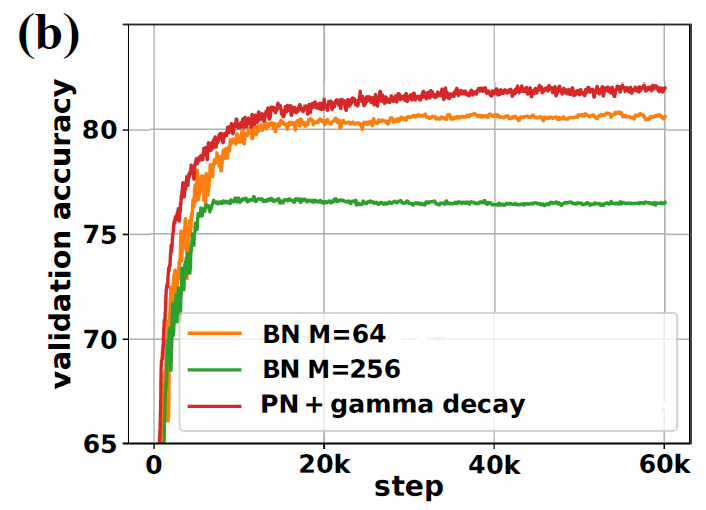#### 2.4.2 $\mu_\mathcal{B},\sigma_\mathcal{B}$的正则能力不同？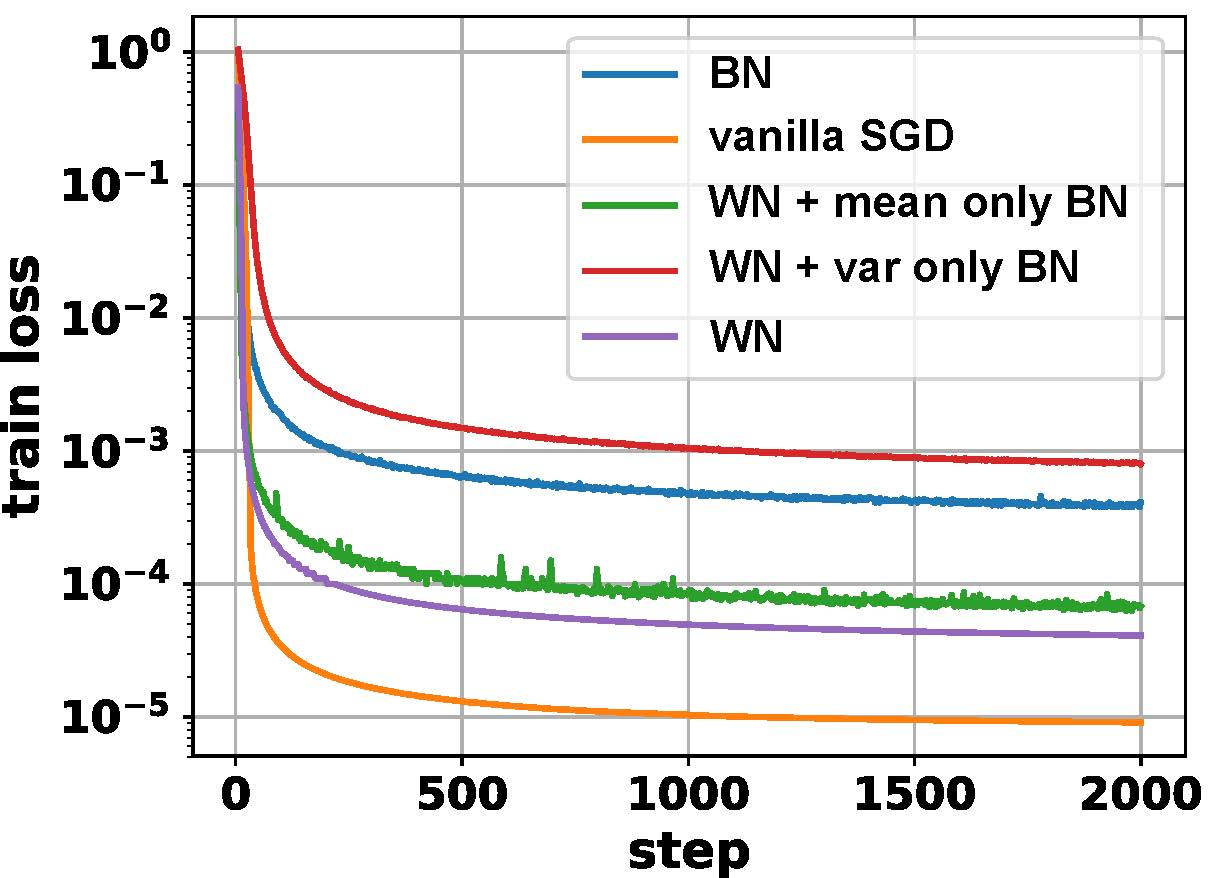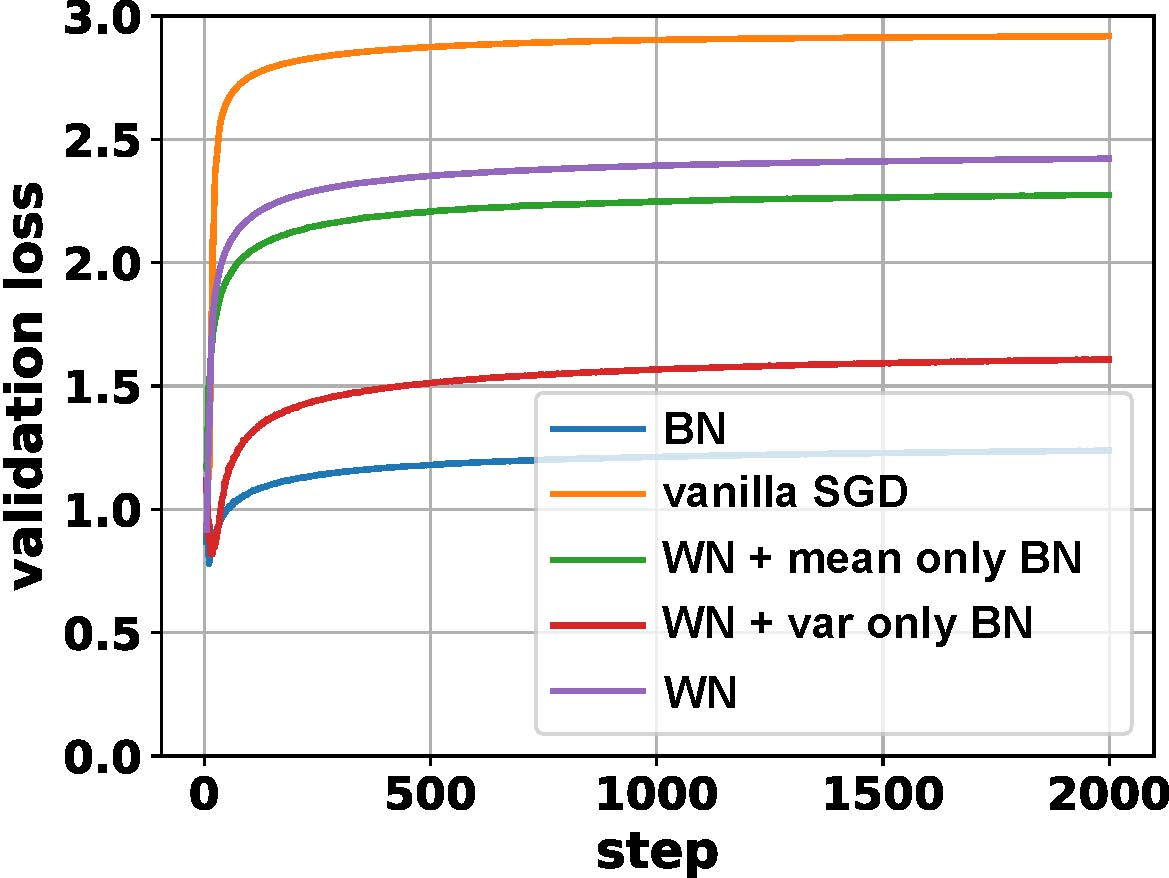#### 2.4.3 BN与批量大小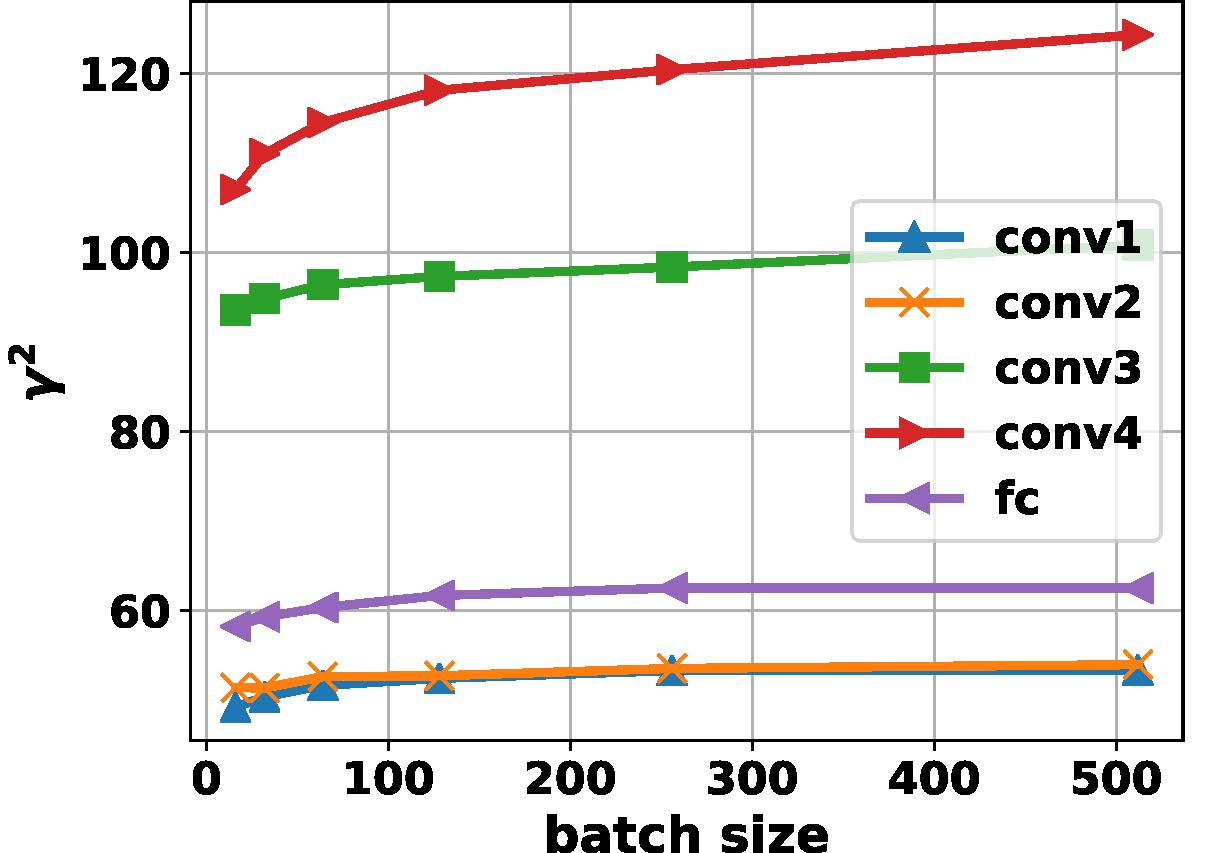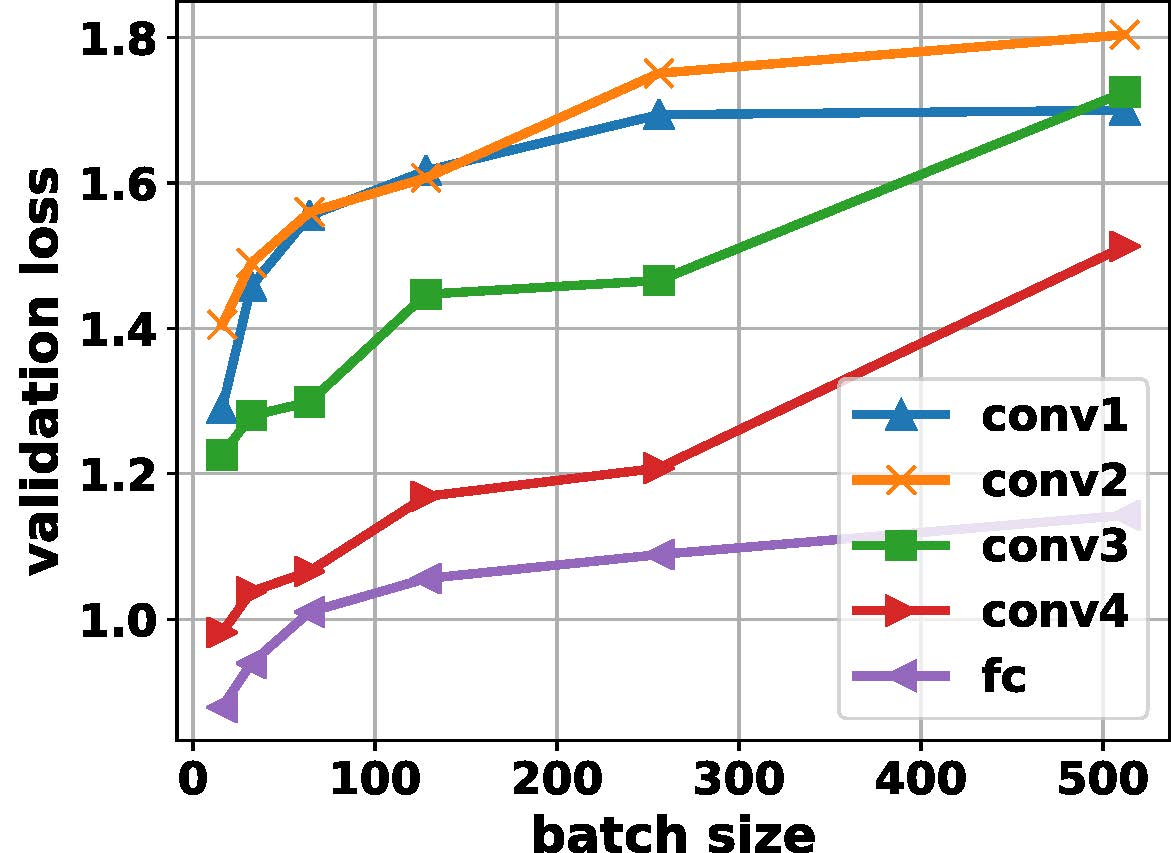#### 2.4.4 BN、WN与Dropout不兼容？

L2正则？：一个直接的想法是在CNN中加入对 $\gamma$ 的正则。实际上这种做法的效果并不好，原因是BN隐含的正则项并不是一个常数而是一个与当前正向与反向参数均有关的值。人工调节很难使得各层均有对应的自适应正则参数，同时引入了复杂的超参系统。这一点在前面也有提到。

BN+Dropout：BN正则项中出现的跟FIM相关的系数与dropout中隐含的正则项类似。因此我们试图采用BN+dropout来额外增加CNN在大批次学习中的正则化强度。下图显示在Cifar-10训练中，若批次由64增加到256时模型的测试误差会显著增加，同时top-1准确率显著降低。但如果在每个BN层后面加入一个dropout层 (ratio=0.125)，这时测试集上的精度甚至会回升到超过原有 $M=64$ 时的准确率。这一现象表面上与BN原文中dropout无法弥补BN效果这一结论有所违背。但实际上，一般而言之前网络训练过程中的批次都较小 ($\sim 32$)，只是在并行计算能力提升之后超大批次的训练才成为可能 (对于Imagenet，批次大小可达60k)。而在小批次训练中BN隐含的正则化能力本身已较强，所以额外dropout引入的正则作用不明显，反而可能带来过大的噪声使得网络无法更好的训练。另外dropout与BN的相对位置也很重要。以往试图利用dropout改善BN的工作将其放在BN之前。但是由于dropout在测试阶段不会带来噪声而BN在测试阶段用的是moving average，导致了网络在测试阶段的统计量有偏差。实际结果也显示dropout放在BN之后效果更好。类似的结论在6等的工作中也有提及。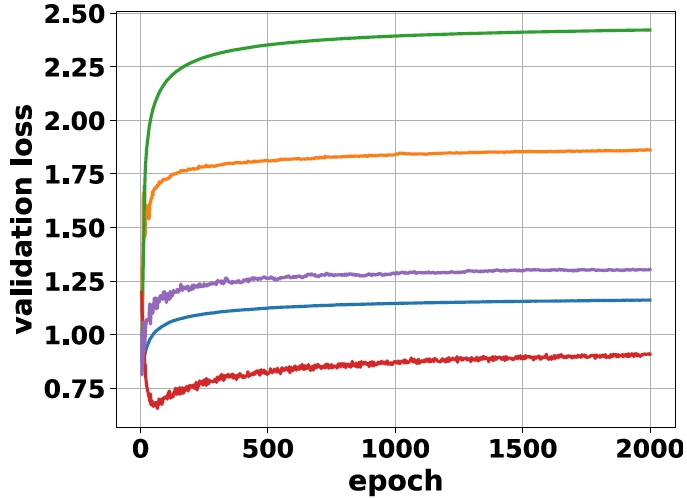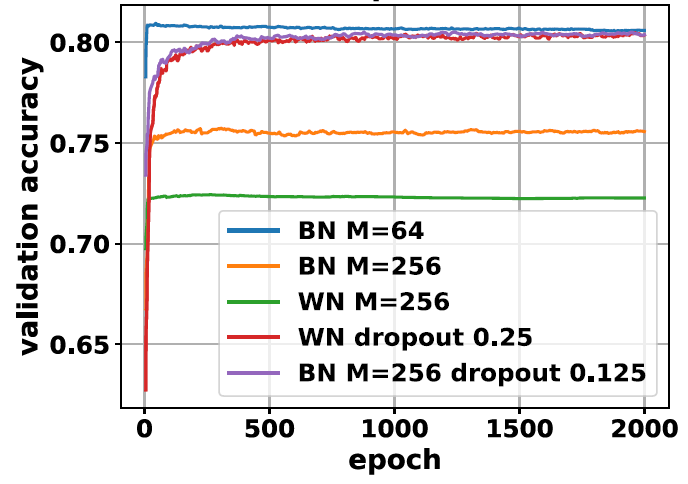WN+Dropout：因为BN在本文的假设条件下可以认为是WN+ $\gamma$ 正则，所以WN的表现加上dropout后也可能得到提升。如上图所示当WN加上dropout (ratio=0.25) 后其top-1准确率由0.73大幅提升到0.80，甚至与BN $M=64$ 训练的结果可相比。因此加入dropout后WN引入了额外的正则化系数使得其具有更好的泛化性能。

1. Sergey Ioffe, Christian Szegedy, “Batch Normalization: Accelerating Deep Network Training by Reducing Internal Covariate Shift”, ICML 2015 ^
2. Ari S. Morcos, David G.T. Barrett, Neil C. Rabinowitz, Matthew Botvinick, “On the importance of single directions for generalization”, ICLR 2018 ^
3. Teye, Mattias, Azizpour, Hossein, and Smith, Kevin. “Bayesian Uncertainty Estimation for Batch Normalized Deep Networks”, ICML 2018 ^
4. Ping Luo, Xinjiang Wang, Wenqi Shao, Zhanglin Peng, “Towards Understanding Regularization in Batch Normalization”, arXiv:1809.00846, 2018 ^
5. Géron, Aurélien. “Hands-on machine learning with Scikit-Learn and TensorFlow: concepts, tools, and techniques to build intelligent systems”, O’Reilly Media Inc., 2017. ^
6. Xiang Li, Shuo Chen, Xiaolin Hu, Jian Yang, “Understanding the Disharmony between Dropout and Batch Normalization by Variance Shift”, arXiv:1801.05134, 2018 ^

## Posts

### 浅谈深度学习：归一化中的正则与泛化

Understanding batch normalization in Deep Learning. This blog was written in Chinese.